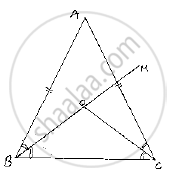# In a δAbc, It is Given that Ab = Ac and the Bisectors of ∠B and ∠C Intersect at O. If M Is A Point on Bo Produced, Prove that ∠Moc = ∠Abc. - Mathematics

In a ΔABC, it is given that AB = AC and the bisectors of ∠B and ∠C intersect at O. If M is a point on BO produced, prove that ∠MOC = ∠ABC.

#### Solution

Given that in , ΔABC,

AB=AC and the bisector of ∠B and ∠C intersect at O and M is
a point on BO produced

We have to prove ∠ MOC=∠ABC

Since,

AB =AC ⇒ΔABC is isosceles ⇒ ∠B=∠C(or) ∠ABC=∠ACB

Now,

BO and CO are bisectors of ∠ABC and ∠ACB  respectively

⇒ABO=∠OBC=∠ACO=∠OB=1/2 ∠ABC=1/2∠ACB ............(1)

We have, in ΔOBC

∠OBC +∠OCB +∠BOC =180°          .............(2)

And also

∠BOC +∠COM =180°             ..................(3)[Straight angle]

Equating (2) and (3)

⇒ ∠OBC+∠OCB+-∠BOC=∠BOC+∠MOC

⇒ ∠OBC+∠OBC=∠MOC           [∵ from (1)]

⇒ 2∠OBBC=∠MOC

⇒2(1/2∠ABC)=∠MOC           [∵from (1)]

⇒ ∠ABC=∠MOC

∴ ∠MOC=∠ABCConcept: Properties of a Triangle
Is there an error in this question or solution?

#### APPEARS IN

RD Sharma Mathematics for Class 9
Chapter 12 Congruent Triangles
Exercise 12.3 | Q 8 | Page 47

Share